# Sets of generalized $H 2$ exponential stability criteria for switched multilayer dynamic neural networks

## Abstract

This paper investigates new sets of generalized $H 2$ exponential stability criteria for switched multilayer dynamic neural networks. These sets of sufficient stability criteria in forms of linear matrix inequality (LMI) and matrix norm are presented, under which switched multilayer dynamic neural networks reduce the effect of external input to a predefined level. The proposed sets of criteria also guarantee exponential stability for switched multilayer dynamic neural networks without external input.

## 1 Introduction

Switched systems are a class of hybrid systems consisting of a family of continuous (or discrete) time subsystems and a logical rule that orchestrates the switching between these subsystems. Switched systems have been extensively researched, and several efforts have been focused on the analysis of switched systems [1, 2]. Recently, switched recurrent neural networks were introduced to represent some complex nonlinear systems efficiently by integrating the theory of switched systems with recurrent neural networks . Switched dynamic neural networks have found applications in the field of artificial intelligence and high speed signal processing [7, 8]. In , some stability criteria for switched dynamic neural networks were studied.

There always exist noise disturbances and model uncertainties in real physical systems. Recently, this has led to an interest in a generalized $H 2$ approach . The generalized $H 2$ approach has been known as a significant concept to examine the stability of various nonlinear dynamical systems. Here, we have the following natural question: Can we obtain a generalized $H 2$ stability criterion for switched dynamic neural networks. This paper provides an answer to this question. To the best of the authors’ knowledge, the generalized $H 2$ analysis of switched dynamic neural networks has not yet been studied in the literature.

In this paper, we propose new sets of generalized $H 2$ exponential stability criteria for switched multilayer dynamic neural networks. The sets of conditions proposed in this paper are a new contribution to the stability evaluation of switched neural networks. The proposed sets of sufficient stability criteria in forms of linear matrix inequality (LMI) and matrix norm guarantee that switched multilayer dynamic neural networks reduce the effect of external input to a predefined level. This paper is organized as follows. In Section 2, new sets of generalized $H 2$ exponential stability criteria are derived. Finally, conclusions are presented in Section 3.

## 2 New sets of generalized $H 2$ exponential stability criteria

Consider the following model of switched multilayer neural networks :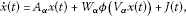(1)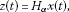(2)

where $x(t)= [ x 1 ( t ) ⋯ x n ( t ) ] T ∈ R n$ is the state vector, $z(t)∈ R p$ is a linear combination of the states, $A=diag{− a 1 ,…,− a n }∈ R n × n$ ($a k >0$, $k=1,…,n$) is the self-feedback matrix, $W∈ R n × n$ and $V∈ R n × n$ are the connection weight matrices, $ϕ(x(t))= [ ϕ 1 ( x ( t ) ) ⋯ ϕ n ( x ( t ) ) ] T : R n → R n$ is the nonlinear function vector satisfying the global Lipschitz condition with Lipschitz constant $L ϕ >0$, $J(t)∈ R n$ is an external input vector, and $H∈ R p × n$ is a known constant matrix. α is a switching signal which takes its values in the finite set $I={1,2,…,N}$. The matrices $( A α , W α , V α , H α )$ are allowed to take values, at an arbitrary time, in the finite set ${( A 1 , W 1 , V 1 , H 1 ),…,( A N , W N , V N , H N )}$. Throughout this paper, we assume that the switching rule α is not known a priori and its instantaneous value is available in real time. Define the indicator function $ξ(t)= ( ξ 1 ( t ) , ξ 2 ( t ) , … , ξ N ( t ) ) T$, where

with $i=1,…,N$. By using this indicator function, the model of the switched multilayer neural networks (1)-(2) can be written as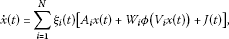(3)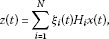(4)

where $∑ i = 1 N ξ i (t)=1$ is satisfied under any switching rules. Let $γ>0$ be a predefined level of disturbance attenuation. In this paper, for a given $κ>0$, we derive sets of criteria such that the switched multilayer neural network (3)-(4) with $J(t)=0$ is exponentially stable ($∥x(t)∥≤ϒexp(−κt)$, where $ϒ>0$) and

$sup t ≥ 0 { exp ( κ t ) z T ( t ) z ( t ) } < γ 2 ∫ 0 ∞ exp(κt) J T (t)J(t)dt,$
(5)

under zero-initial conditions for all nonzero $J(t)∈ L 2 [0,∞)$, where $L 2 [0,∞)$ is the space of square integrable vector functions over $[0,∞)$.

A set of generalized $H 2$ exponential stability criterion of the switched multilayer neural network (3)-(4) is derived in the following theorem.

Theorem 1 For given$γ>0$and$κ>0$, the switched multilayer neural network (3)-(4) is generalized$H 2$exponentially stable if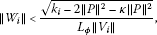(6)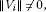(7)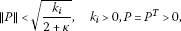(8)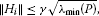(9)

for$i=1,…,N$, where$λ min (⋅)$is the minimum eigenvalue of the matrix and P satisfies the Lyapunov inequality$A i T P+P A i <− k i I$.

Proof We consider the Lyapunov function $V(t)=exp(κt) x T (t)Px(t)$. The time derivative of the function along the trajectory of (3) satisfies

$V ˙ (t)< ∑ i = 1 N ξ i (t)exp(κt) { − x T ( t ) [ k i − κ P ] x ( t ) + 2 x T ( t ) P W i ϕ ( V i x ( t ) ) + 2 x T ( t ) P J ( t ) } .$
(10)

Applying Young’s inequality , we have $2 x T (t)P W i ϕ( V i x(t))≤ x T (t)PPx(t)+ ϕ T ( V i x(t)) W i T W i$, $ϕ( V i x(t))≤ ∥ P ∥ 2 ∥ x ( t ) ∥ 2 + L ϕ 2 ∥ W i ∥ 2 ∥ V i ∥ 2 ∥ x ( t ) ∥ 2$ and $2 x T (t)PJ(t)≤ x T (t)P P T x(t)+ J T (t)J(t)≤ ∥ P ∥ 2 ∥ x ( t ) ∥ 2 + ∥ J ( t ) ∥ 2$. Substituting these inequalities into (10), we have

$V ˙ ( t ) < ∑ i = 1 N ξ i ( t ) exp ( κ t ) { − [ k i − ( 2 + κ ) ∥ P ∥ 2 − L ϕ 2 ∥ W i ∥ 2 ∥ V i ∥ 2 ] ∥ x ( t ) ∥ 2 + ∥ J ( t ) ∥ 2 } = − ∑ i = 1 N ξ i ( t ) exp ( κ t ) [ k i − ( 2 + κ ) ∥ P ∥ 2 − L ϕ 2 ∥ W i ∥ 2 ∥ V i ∥ 2 ] ∥ x ( t ) ∥ 2 + ∑ i = 1 N ξ i ( t ) exp ( κ t ) ∥ J ( t ) ∥ 2 .$
(11)

If the following condition is satisfied,

$k i −(2+κ) ∥ P ∥ 2 − L ϕ 2 ∥ W i ∥ 2 ∥ V i ∥ 2 >0,$
(12)

for $i=1,…,N$, we have

$V ˙ ( t ) < ∑ i = 1 N ξ i ( t ) exp ( κ t ) ∥ J ( t ) ∥ 2 = exp ( κ t ) ∥ J ( t ) ∥ 2 .$
(13)

The following inequalities

$∥ W i ∥ 2 < k i − ( 2 + κ ) ∥ P ∥ 2 L ϕ 2 ∥ V i ∥ 2 , ∥ P ∥ 2 < k i 2 + κ ,$
(14)

for $i=1,…,N$, imply the condition (12). Thus, we obtain (6) and (7). Under the zero-initial condition, we have $V(t) | t = 0 =0$ and $V(t)≥0$. Define

$Φ(t)=V(t)− ∫ 0 t exp(κσ) J T (σ)J(σ)dσ.$
(15)

Then, for any nonzero $J(t)$, we obtain

$Φ ( t ) = V ( t ) − V ( t ) | t = 0 − ∫ 0 t exp ( κ σ ) J T ( σ ) J ( σ ) d σ = ∫ 0 t [ V ˙ ( σ ) − exp ( κ σ ) J T ( σ ) J ( σ ) ] d σ .$

From (13), we have $Φ(t)<0$. It means

$V(t)< ∫ 0 t exp(κσ) J T (σ)J(σ)dσ.$

The condition (9) implies

$exp ( κ t ) z T ( t ) z ( t ) = ∑ i = 1 N ξ i ( t ) exp ( κ t ) x T ( t ) H i T H i x ( t ) ≤ ∑ i = 1 N ξ i ( t ) exp ( κ t ) ∥ H i ∥ 2 ∥ x ( t ) ∥ 2 ≤ γ 2 ∑ i = 1 N ξ i ( t ) exp ( κ t ) λ min ( P ) ∥ x ( t ) ∥ 2 ≤ γ 2 ∑ i = 1 N ξ i ( t ) exp ( κ t ) x T ( t ) P x ( t ) = γ 2 V ( t ) < γ 2 ∫ 0 t exp ( κ σ ) J T ( σ ) J ( σ ) d σ ≤ γ 2 ∫ 0 ∞ exp ( κ σ ) J T ( σ ) J ( σ ) d σ .$
(16)

Taking the supremum over $t>0$ leads to (5). This completes the proof. □

Corollary 1 When$J(t)=0$, the conditions (6)-(9) ensure that the switched multilayer neural network (3)-(4) is exponentially stable.

Proof When $J(t)=0$, from (13), $V ˙ (t)<0$ for all $x(t)≠0$. Thus, for any $t≥0$, it implies that

$exp(κt) ∥ x ( t ) ∥ 2 λ min (P)≤exp(κt) x T (t)Px(t)=V(t)
(17)

Finally, we have

$∥ x ( t ) ∥ < x T ( 0 ) P x ( 0 ) λ min ( P ) exp ( − κ 2 t ) .$
(18)

This completes the proof. □

In the next theorem, we find a new set of LMI criteria for the generalized $H 2$ exponential stability of the switched multilayer neural network (3)-(4). This set of LMI criteria can be facilitated readily via standard numerical algorithms [21, 22].

Theorem 2 For given level$γ>0$and$κ>0$, the switched multilayer neural network (3)-(4) is generalized$H 2$exponentially stable if there exist a positive symmetric matrix P and a positive scalar ϵ such that(19)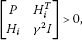(20)

for$i=1,…,N$.

Proof Consider the Lyapunov function $V(t)=exp(κt) x T (t)Px(t)$. Applying Young’s inequality , we have $ϵ[ L ϕ 2 x T (t) V i T V i x(t)− ϕ T ( V i x(t))ϕ( V i x(t))]≥0$. By using this inequality, the time derivative of $V(t)$ along the trajectory of (3) is

$V ˙ ( t ) = ∑ i = 1 N ξ i ( t ) exp ( κ t ) { x T ( t ) [ A i T P + P A i + κ P ] x ( t ) + 2 x T ( t ) P W i ϕ ( V i x ( t ) ) + 2 x T ( t ) P J ( t ) } ≤ ∑ i = 1 N ξ i ( t ) exp ( κ t ) { x T ( t ) [ A i T P + P A i + κ P ] x ( t ) + 2 x T ( t ) P W i ϕ ( V i x ( t ) ) + 2 x T ( t ) P J ( t ) + ϵ [ L ϕ 2 x T ( t ) V i T V i x ( t ) − ϕ T ( V i x ( t ) ) ϕ ( V i x ( t ) ) ] } = ∑ i = 1 N ξ i ( t ) exp ( κ t ) [ x ( t ) ϕ ( V i x ( t ) ) J ( t ) ] T [ A i T P + P A i + κ P + ϵ L ϕ 2 V i T V i P W i P W i T P − ϵ I 0 P 0 − I ] × [ x ( t ) ϕ ( V i x ( t ) ) J ( t ) ] + ∑ i = 1 N ξ i ( t ) exp ( κ t ) J T ( t ) J ( t ) .$
(21)

If the LMI (19) is satisfied, we have

$V ˙ ( t ) < ∑ i = 1 N ξ i ( t ) exp ( κ t ) J T ( t ) J ( t ) = exp ( κ t ) J T ( t ) J ( t ) .$
(22)

Under the zero-initial condition, one has $V(t) | t = 0 =0$ and $V(t)≥0$. Define

$Φ(t)=V(t)− ∫ 0 t exp(κσ) J T (σ)J(σ)dσ.$
(23)

Then, for any nonzero $J(t)$, we obtain

$Φ ( t ) = V ( t ) − V ( t ) | t = 0 − ∫ 0 t exp ( κ σ ) J T ( σ ) J ( σ ) d σ = ∫ 0 t [ V ˙ ( σ ) − exp ( κ σ ) J T ( σ ) J ( σ ) ] d σ .$

From (22), we have $Φ(t)<0$. It means

$V(t)< ∫ 0 t exp(κσ) J T (σ)J(σ)dσ.$

The LMI (20) implies

$exp ( κ t ) z T ( t ) z ( t ) = ∑ i = 1 N ξ i ( t ) exp ( κ t ) x T ( t ) H i T H i x ( t ) < γ 2 ∑ i = 1 N ξ i ( t ) exp ( κ t ) x T ( t ) P x ( t ) = γ 2 V ( t ) < γ 2 ∫ 0 t exp ( κ σ ) J T ( σ ) J ( σ ) d σ ≤ γ 2 ∫ 0 ∞ exp ( κ σ ) J T ( σ ) J ( σ ) d σ .$
(24)

Taking the supremum over $t>0$ leads to (5). This completes the proof. □

Corollary 2 When$J(t)=0$, a set of LMI conditions (19)-(20) ensure that the switched multilayer neural network (3)-(4) is exponentially stable.

Proof When $J(t)=0$, from (22), we have

$V ˙ (t)<0,∀x(t)≠0.$
(25)

Thus, for any $t≥0$, it implies that

$exp(κt) ∥ x ( t ) ∥ 2 λ min (P)≤exp(κt) x T (t)Px(t)=V(t)
(26)

Finally, we have

$∥ x ( t ) ∥ < x T ( 0 ) P x ( 0 ) λ min ( P ) exp ( − κ 2 t ) .$
(27)

This completes the proof. □

Example 1 Consider the switched neural network (3)-(4), where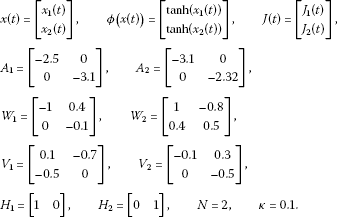Applying Theorem 2 with $γ=0.52$, we obtain

$P= [ 3.7405 0.0474 0.0474 3.7059 ] ,ϵ=6.2769.$

The switching signal $α∈{1,2}$ is given by

$α={ 1 , 2 k ≤ t ≤ 2 k + 1 , k ∈ Z , 2 , otherwise ,$

where Z is the whole set of nonnegative integers. Figure 1 shows state trajectories when $x(0)= [ − 1.5 0.5 ] T$ and $J i (t)$ ($i=1,2$) is a white noise.

## 3 Conclusion

In this paper, we have proposed new sets of generalized $H 2$ exponential stability criteria for switched multilayer dynamic neural networks. These sets of sufficient stability criteria are represented by matrix norm and LMI. The proposed sets of criteria ensured that switched multilayer dynamic neural networks attenuate the effect of external input on the state vector. These sets of criteria also guaranteed exponential stability for switched multilayer dynamic neural networks when there is no external input.

## References

1. 1.

Lee S, Kim T, Lim J: A new stability analysis of switched systems. Automatica 2000, 36(6):917–922. 10.1016/S0005-1098(99)00208-3

2. 2.

Daafouz J, Riedinger P, Iung C: Stability analysis and control synthesis for switched systems: a switched Lyapunov function approach. IEEE Trans. Autom. Control 2002, 47(11):1883–1887. 10.1109/TAC.2002.804474

3. 3.

Huang H, Qu Y, Li H: Robust stability analysis of switched Hopfield neural networks with time-varying delay under uncertainty. Phys. Lett. A 2005, 345: 345–354. 10.1016/j.physleta.2005.07.042

4. 4.

Yuan K, Cao J, Li H: Robust stability of switched Cohen-Grossberg neural networks with mixed time-varying delays. IEEE Trans. Syst. Man Cybern., Part B, Cybern. 2006, 36(6):1356–1363.

5. 5.

Li P, Cao J: Global stability in switched recurrent neural networks with time-varying delay via nonlinear measure. Nonlinear Dyn. 2007, 49(1–2):295–305. 10.1007/s11071-006-9134-9

6. 6.

Lou X, Cui B: Delay-dependent criteria for robust stability of uncertain switched Hopfield neural networks. Int. J. Autom. Comput. 2007, 4(3):304–314. 10.1007/s11633-007-0304-0

7. 7.

Tsividis, Y: Switched neural networks. US Patent 4873661, 1989

8. 8.

Brown T: Neural networks for switching. IEEE Commun. Mag. 1989, 27(11):72–81.

9. 9.

Grigoriadis K, Watson J:Reduced-order $H ∞$ and $L 2 − L ∞$ filtering via linear matrix inequalities. IEEE Trans. Aerosp. Electron. Syst. 1997, 33: 1326–1338.

10. 10.

Watson J, Grigoriadis K: Optimal unbiased filtering via linear matrix inequalities. Syst. Control Lett. 1998, 35: 111–118. 10.1016/S0167-6911(98)00042-5

11. 11.

Palhares R, Peres P: Robust filtering with guaranteed energy-to-peak performance - an LMI approach. Automatica 2000, 36: 851–858. 10.1016/S0005-1098(99)00211-3

12. 12.

Gao H, Wang C:Robust $L 2 − L ∞$ filtering for uncertain systems with multiple time-varying state delays. IEEE Trans. Circuits Syst. I, Fundam. Theory Appl. 2003, 50: 594–599. 10.1109/TCSI.2003.809816

13. 13.

Gao H, Wang C:Delay-dependent robust $H ∞$ and $L 2 − L ∞$ filtering for a class of uncertain nonlinear time-delay systems. IEEE Trans. Autom. Control 2003, 48: 1661–1666. 10.1109/TAC.2003.817012

14. 14.

Mahmoud M:Resilient $L 2 − L ∞$ filtering of polytopic systems with state delays. IET Control Theory Appl. 2007, 1: 141–154. 10.1049/iet-cta:20045281

15. 15.

Qiu J, Feng G, Yang J: New results on robust energy-to-peak filtering for discrete-time switched polytopic linear systems with time-varying delay. IET Control Theory Appl. 2008, 2(9):795–806. 10.1049/iet-cta:20070361

16. 16.

Zhou Y, Li J: Energy-to-peak filtering for singular systems: the discrete-time case. IET Control Theory Appl. 2008, 2(9):773–781. 10.1049/iet-cta:20070432

17. 17.

Ahn C:$L 2 − L ∞$ nonlinear system identification via recurrent neural networks. Nonlinear Dyn. 2010, 62: 543–552. 10.1007/s11071-010-9741-3

18. 18.

Ahn C:$L 2 − L ∞$ chaos synchronization. Prog. Theor. Phys. 2010, 123(3):421–430. 10.1143/PTP.123.421

19. 19.

Ahn C:An answer to the open problem of $L 2 − L ∞$ synchronization for time-delayed chaotic systems. Eur. Phys. J. Plus 2012., 127(2): Article ID 22

20. 20.

Arnold V: Mathematical Methods of Classical Mechanics. Springer, New York; 1989.

21. 21.

Boyd S, Ghaoui LE, Feron E, Balakrishinan V: Linear Matrix Inequalities in Systems and Control Theory. SIAM, Philadelphia; 1994.

22. 22.

Gahinet P, Nemirovski A, Laub AJ, Chilali M: LMI Control Toolbox. The Mathworks, Natic; 1995.

## Acknowledgements

This research was supported by the MKE (The Ministry of Knowledge Economy), Korea, under the CITRC (Convergence Information Technology Research Center) support program (NIPA-2012-H0401-12-1007) supervised by the NIPA (National IT Industry Promotion Agency).

## Author information

Authors

### Corresponding author

Correspondence to Choon Ki Ahn.

### Competing interests

The authors declare that they have no competing interests.

### Authors’ contributions

All authors contributed equally and significantly in writing this paper. All authors read and approved the final manuscript.

## Authors’ original submitted files for images

Below are the links to the authors’ original submitted files for images.

## Rights and permissions

Reprints and Permissions

Ahn, C.K., Lee, Y.S. Sets of generalized $H 2$ exponential stability criteria for switched multilayer dynamic neural networks. Adv Differ Equ 2012, 150 (2012). https://doi.org/10.1186/1687-1847-2012-150